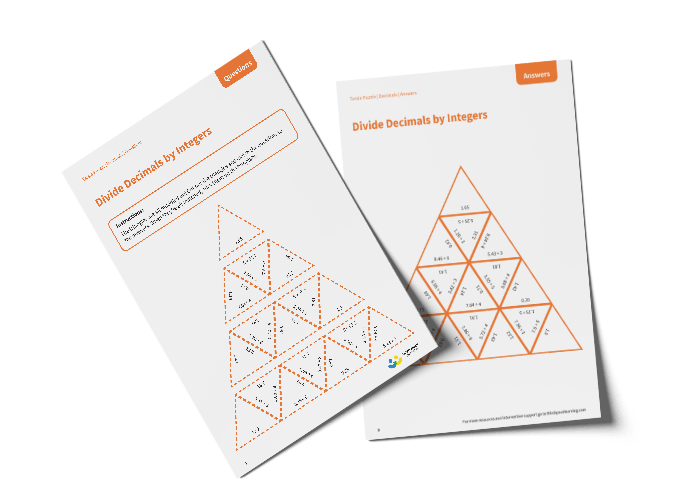# Tarsia Puzzle Divide Decimals by Integers (Year 6)

The triangles in the tarsia puzzle have all been mixed up. In this dividing decimals by integer tarsia puzzle, pupils will need to match the division question to the correct answer to create one large triangle.

This Year 6 divide decimals by integers tarsia puzzle worksheet contains:

• One question sheet
• One template sheet

Year 6

Decimals